# Memory utilization

Depending on how the IQANdesign application is built, there can be significant differences between memory and cycle utilization for the exact same functionality.

This knowledge base article looks at a few minimal examples to illustrate differences in memory utilization.

## Utilization example A

Consider a function that:

• checks that two sensor values are below a fixed threshold
• has an override that can activate the function even if the condition for the sensors aren't met

This is a relatively inefficient Qcode implementation:

`overrideVariable := OverridesensorA_Low := SensorA < 50sensorB_Low := SensorB < 50if (SensorA_Low and sensorB_Low) or overrideVariable then Result:=True`

It does however have the advantage that when measuring, each condition has a line of its own: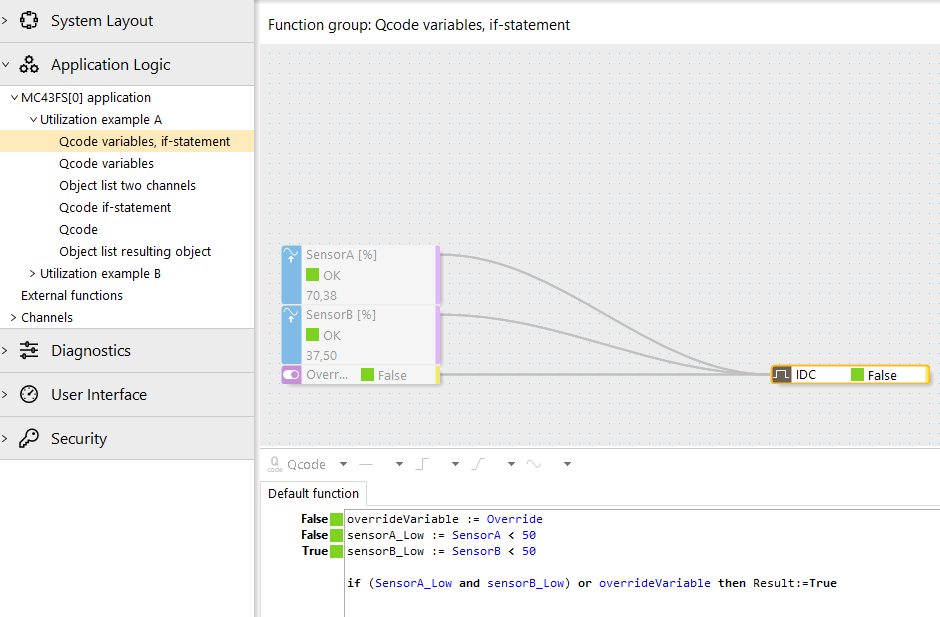For a slightly more efficient implementation, the if-statement can be omitted, assigning Result directly.

`overrideVariable := OverridesensorA_Low := SensorA < 50sensorB_Low := SensorB < 50Result:= (SensorA_Low and sensorB_Low) or overrideVariable`

A more efficient Qcode expression can be achieved by omitting the variables that hold intermediate results:

`Result:= (SensorA < 50 and SensorB < 50) or Override`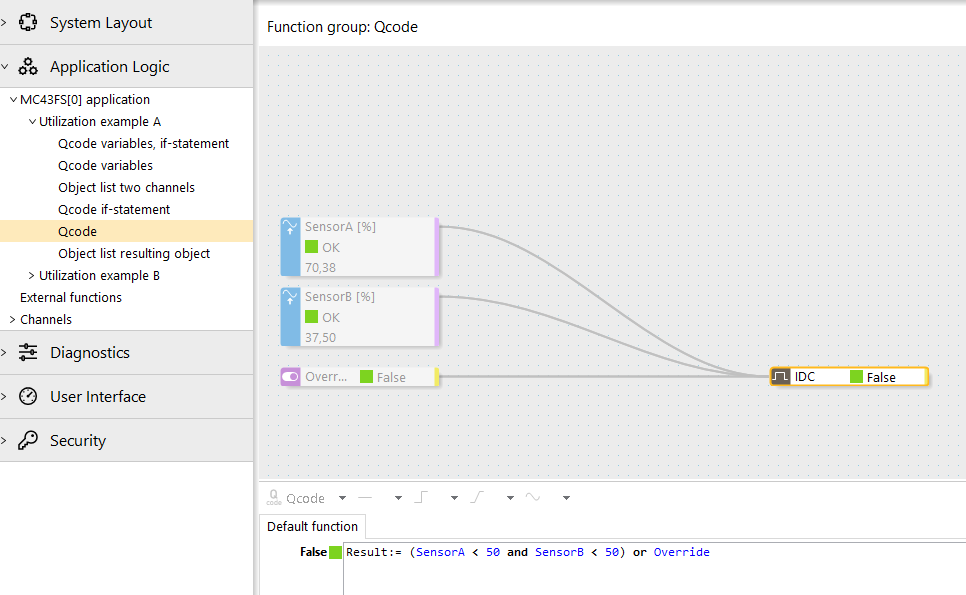A drawback of this single line is that it can be harder to see the individual conditions. Here a classic object list can both help, and reduce memory utilization further.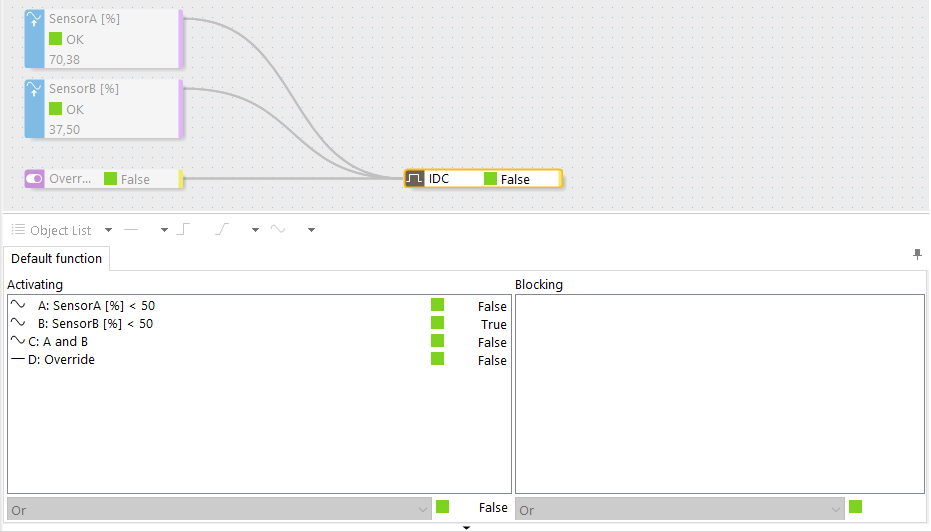The difference in RAM memory utilization can be seen i the project statistics.

Estimate calculated in IQANdesign 5.06: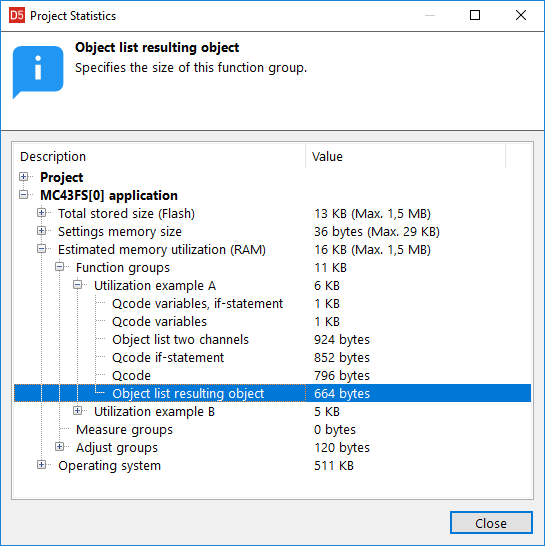## Utilization example B

Consider a function that:

• checks that a sensor value (e.g. speed) is close to zero
• at the same time checks the sensor status, to handle the event that the value zero might be due to a sensor fault

A relatively inefficient Qcode implementation could look like this:

```//check that movement is stoppedspeedIsLow := Speed < 1speedSensorOK := StatusOf(Speed) = stOKif speedIsLow and speedSensorOK then  Result := Trueelse  Result := Falseendif
```

That can easily be simplified to:

`//check that movement is stoppedspeedIsLow := Speed < 1speedSensorOK := StatusOf(Speed) = stOKResult := speedIsLow and speedSensorOK`

A more compact implementation that saves memory would be:

```//check that movement is stoppedResult := Speed < 1 and StatusOf(Speed) = stOK
```

In this example, an object list would be the most efficient: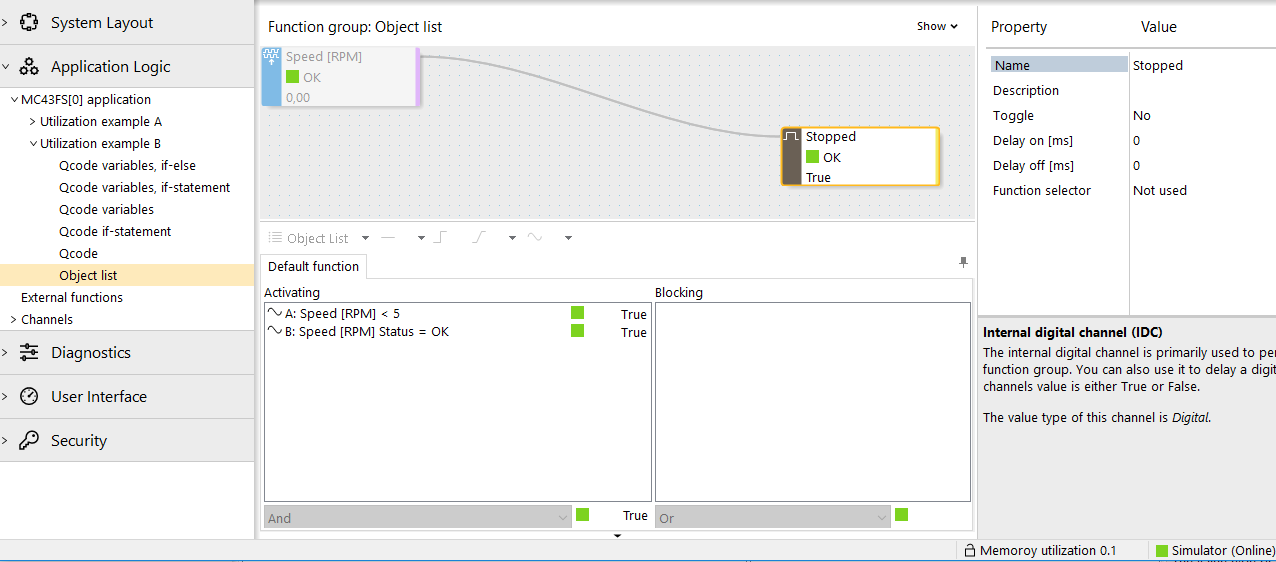The difference in RAM memory utilization can be seen i the project statistics.

Estimate calculated in IQANdesign 5.06: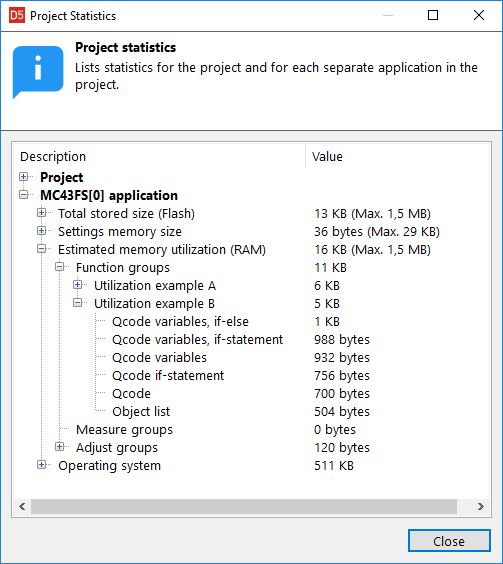For further detail, see attached example file:

These specific MC4x memory utilization estimates with IQANdesign 5.06. The system information channel for MC4x will show the actual total memory utilization.

Note that these examples are put in individual function groups for easy comparison in project statistics. Channel scope protected is used to eliminate the impact of FGI channels, but each FG:s in itself also contributes to the utilization seen here.

Optimizations in future 6.x versions may improve RAM utilization.